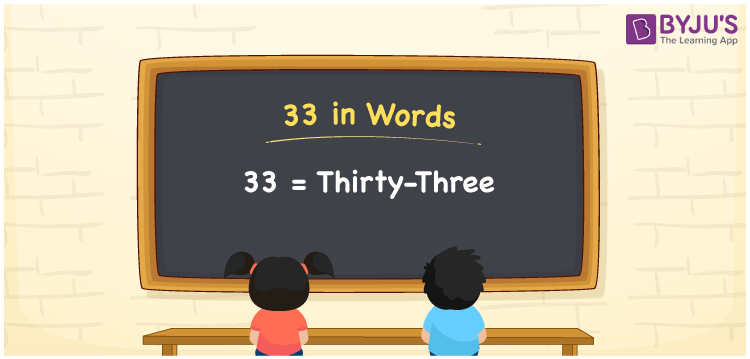# 33 in Words

The number 33 in words is expressed as Thirty-Three. We know that 33 is a two-digit number that has two place values, ones and tens. English is the most commonly used language and it is used to represent any number in words. 33 is a cardinal number since it denotes a specific quantity. Learn how to write 33 in words in this article.

 33 in words Thirty-Three Thirty-Three in numerical form 33

## 33 in English Words

English is the most commonly used language to write any numbers in words. Hence, students must learn how to read and write numbers in the English language for easy and better learning. Therefore, the number 33 in English is spelt as “Thirty-Three”.## How to Write 33 in Words?

The number 33 has two digits. The place value of 3 is at tens place and 3 is at ones place.

Hence, the number 33 can be represented using the place value method.

 Tens Ones 3 3

Hence, we can write the expanded form as:

3 × Ten + 3 × One

= 3 × 10 + 3 × 1

= 30 + 3

= 33

= Thirty-Three

Therefore, 33 in words is written as Thirty-Three.

Interesting way of writing 33 in words

3 = Three

30 = Thirty

30 + 3= 33

Thirty + Three = Thirty-Three

Hence, the word form of the number 33 is Thirty-Three.

33 is a natural number that is the successor of 32 and the predecessor of 34

• 33 in words – Thirty-Three
• Is 33 an odd number? – Yes
• Is 33 an even number? – No
• Is 33 a perfect square number? – No
• Is 33 a perfect cube number? – No
• Is 33 a prime number? – No
• Is 33 a composite number? – Yes

## Frequently Asked Questions on 33 in Words

Q1

### How to write 33 in words?

33 in words is written as Thirty-Three.
Q2

### Is 33 an odd number?

Yes, 33 is an odd number.
Q3

### Determine the value of 20 + 13 and write the answer in words.

20 + 13 gives 33. The number 33 in words is expressed as Thirty-Three.